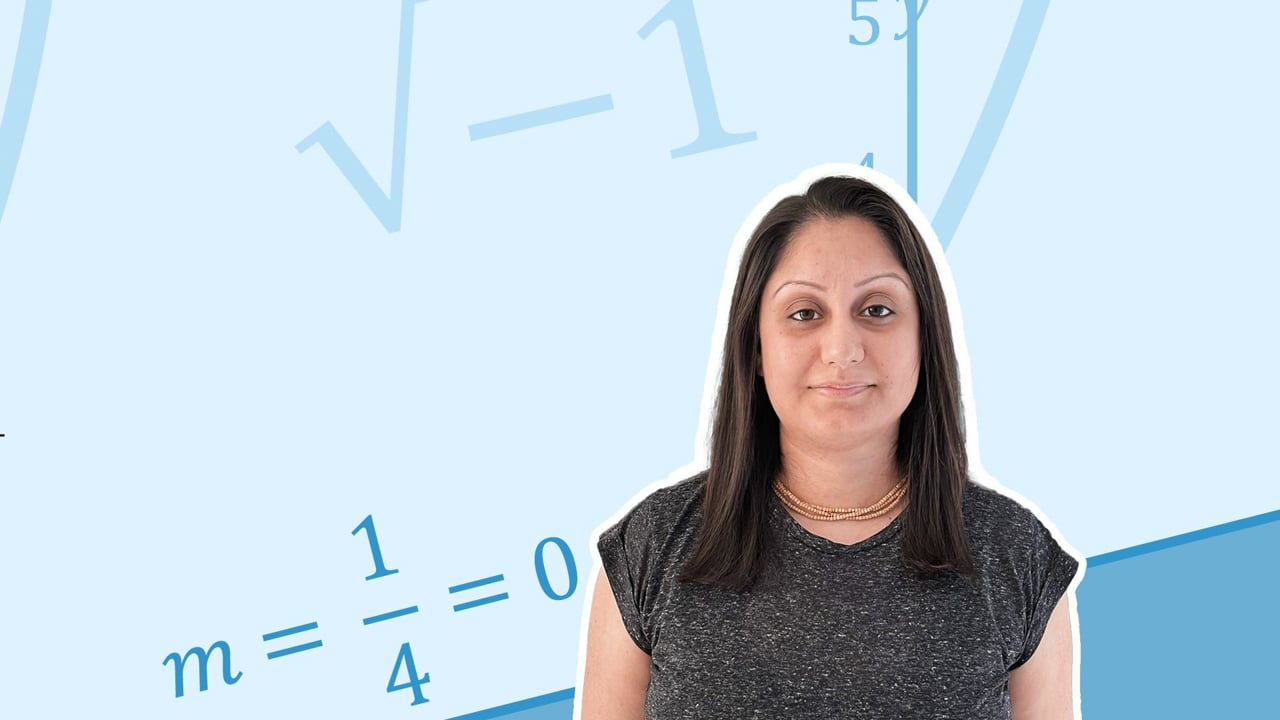Chapter overviewMaths

Exam board

AQA

Number

Algebra

Graphs

Ratio proportion and rates of change

Shapes and area

Angles and geometry

Trigonometry

Probability

Statistics

Maths

# Multiplying and dividing algebraic expressions0%

Summary

# Multiplying and dividing algebraic expressions

## ​​In a nutshell

Algebraic terms can be multiplied or divided. If the variables are the same, the laws of indices can be used. When multiplying, different variables can be written together without the mulitplication sign between them. For dividing, variables can be written as a fraction. Numbers can be multiplied or divided as usual.

## Multiplying

To multiply two terms, multiply numbers as normal and add the powers on the (same) variables using the rule:

$x^a \times x^b = x^{a+b}$

This rule can only be applied if variables are the same. If variables are different, write them together, omitting the multiplication sign. The number on the power tells you how many times that variable has been multiplied together.

##### Examples

\begin {aligned}a \times 0 &= 0 \\a \times 1 &= a \\a \times a &= a^2 \\a^2 \times a^3 &= a^5 \\2a^4 \times 3a^5 &= 6a^9 \\ a \times b &= ab \\ 2a^2 \times 5ab &=10a^3b \\\end {aligned}​​

## Dividing

To divide two terms, divide the numbers as normal and subtract the powers on the (same) variables using the rule:

$x^a \div x^b = x^{a-b}$

This rule can only be applied if  the variables are the same. If the variables are different, write them as a fraction.

##### Examples

\begin {aligned}\frac {6a} 3 &= 2a \\\frac {12x} {2x} &= 6 \\\frac {15x^2} {3x} &= 5x \\ \frac {10a} {2b} &= \frac {5a} b \\ \frac {27a^3b^2c} {3a^2c} &= 9ab^2\end {aligned}

## Want to find out more? Check out these other lessons!

Multiplying and dividing algebraic expressions

FAQs

• Question: How do you multiply or divide variables with different letters in algebra?

Answer: When multiplying variables with different letters, the variables can be written together without the mulitplication sign between them, for example, 2x x 3y = 6xy. For dividing variables with different letters, variables can be written as a fraction, for example, 6x / 2y = 3x/y.

• Question: How do you divide algrabraic expressions?

Answer: To divide algebraic expressions, use the laws of indices if the variables are the same. The rule is a^m / a^n = a^(m-n), when you are dividing, subtract the powers. E.g. a^5 / a^2 = a^3.

• Question: How do you multiply algebraic expressions?

Answer: To multiply algebraic expressions, use the laws of indices if the variables are the same. The rule is a^m x a^n = a^(m+n), so when multiplying two terms, add the powers. For example, a^2 x a^3 = a^5.

Theory

Exercises